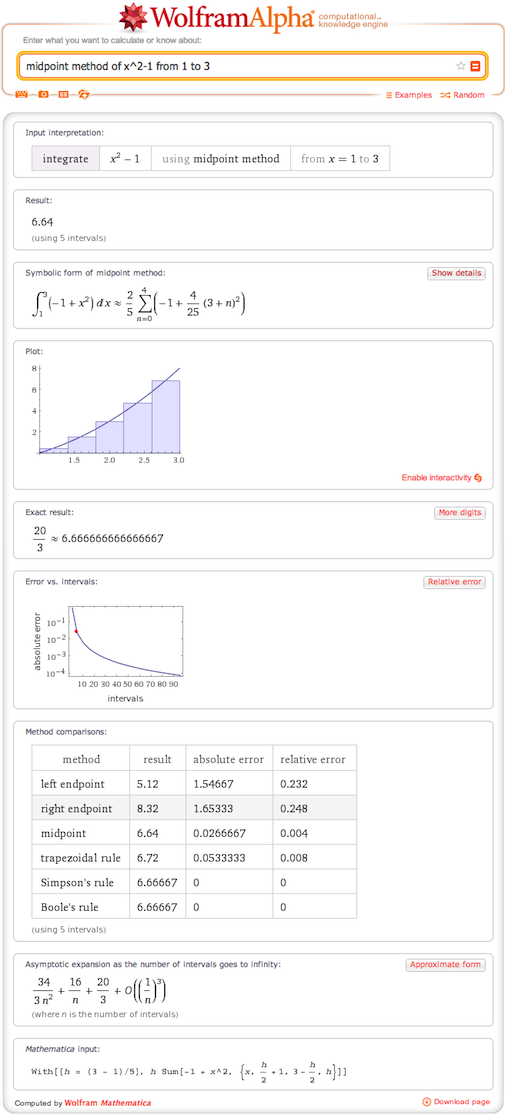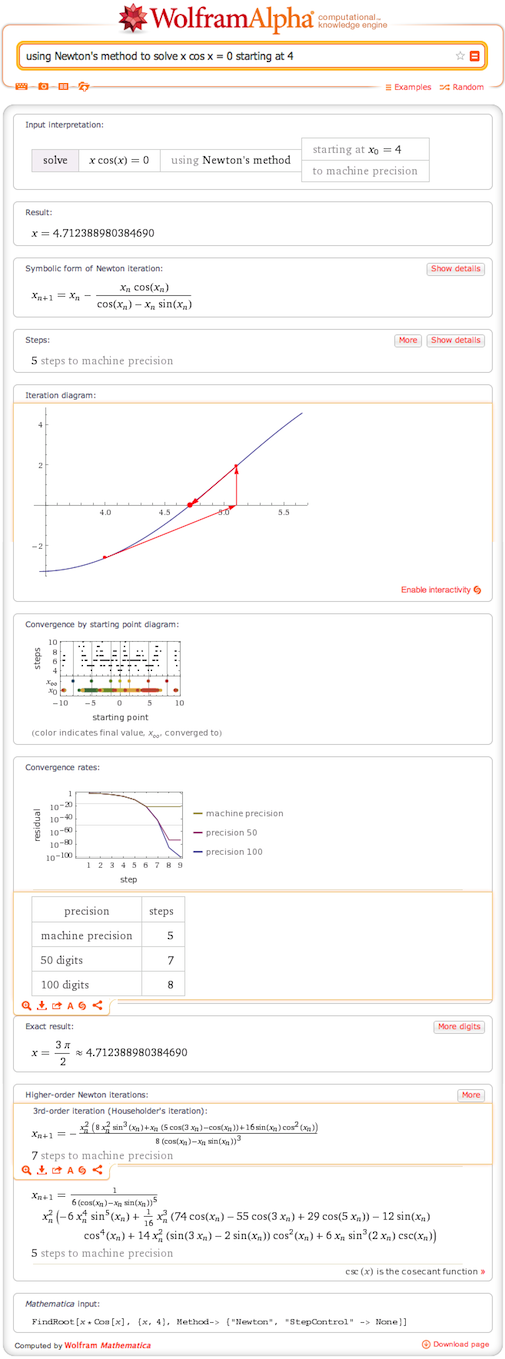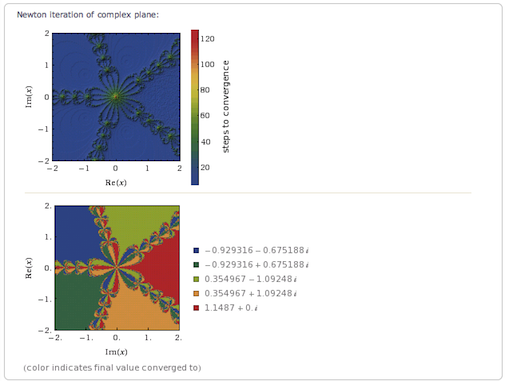# Numerical Methods: Root Finding

June 25, 2013 —Comments Off

Last year we greatly expanded our step-by-step functionality for mathematical problems in Wolfram|Alpha. These tools can be a great aid for students to understand the methods of solving integrals and equations symbolically. But what if we are not looking for a symbolic result? What if we need a numerical approximation? For example, we might be looking at an integral or differential equation that cannot be solved in a closed form, or we might just want to find where an equation intercepts the axis.

Over the centuries, mathematicians have developed many ways of approximating the numerical answer to an integral or finding the root of an equation. Now you can access the same methods directly in Wolfram|Alpha.

Integration is where many of us have encountered numerical methods. The simple approximations are used in high school and introductory college classes to form a starting point for more advanced methods of solving integrals.

One of the most basic methods for approximating an integral is the Riemann sum. In this method you divide the region you are integrating over into a number of intervals. You can then find the value of the function you are integrating, called the integrand, at some point within each interval. By multiplying those values by the width of each interval and then summing, you can get an approximation to the value of the integral. A basic example of this is the midpoint method (midpoint method of x^2-1 from 1 to 3), where you calculate the value at the center of each interval.You can look at the steps to examine each step of the calculation or examine a diagram to see how the interval converges with each step.

The fastest root-finding method we have included is Newton’s method, which uses the derivative at a point on the curve to calculate the next point on the way to the root. Accuracy with this method increases as the square of the number of iterations. Here is an example using Newton’s method to solve x cos x = 0 starting at 4.In both of our examples, we illustrate the symbolic code behind these methods and the steps taken to reach the solution. For Newton’s method, we have also included data on higher-order versions of the method. These versions include more correction terms than the normal method, which increases the calculation’s complexity while increasing the speed of convergence.

A final root-finding method we have included is the secant method (solve x cos x using secant method). This takes two points and builds a secant line through them. This method is an approximation to Newton’s method and is somewhat less efficient in theory, though since it doesn’t require finding the derivative, it may be faster in practice for certain problems.

The starting point chosen for a root-finding calculation is very important both in terms of the solution found and the iterations required for convergence. In cases where there are multiple solutions, the starting point or points determine which solution you will arrive at. Perhaps equally important is how long it takes for a calculation to converge. Our starting points diagram graphically illustrates both these features.

In cases where there are complex roots, the starting value plot for Newton’s method can result in extraordinary fractal patterns. For example, using Newton’s method solve x^5-2 starting at 2 i generates:Nepal is an undeveloped country .so in nepal, i want to help to teach and papularise mathematics .but i need some tecnic about Geometry

Posted by mukesh khatiwada June 25, 2013 at 10:36 am

You can look at the steps to examine each step of the calculation or examine a diagram to see how the interval converges with each step.

Posted by topdiablo3 June 28, 2013 at 2:55 am

Excellent!! I am taking full advantage of these blogs while teaching online

Posted by Wasim Zahid July 1, 2013 at 6:44 am

I just love this new features, will show this to my sister, will appreciate it as she is math teacher 🙂

Posted by Christine Derrel August 27, 2013 at 7:18 am

I use this all the time and must say is has helped me a lot in my teaching ways, Very simple but very effective.

Posted by Katie fitch May 11, 2014 at 12:29 pm

Hi Jason, very professional guide

Posted by Levitt Jean June 11, 2014 at 11:30 am

Great guide, Jason. Will bookmark it for my students.

Posted by Kimberly Gibbs June 27, 2014 at 4:15 am

Very interesting. Thank you for making it so clear.

Posted by Aubrey November 24, 2015 at 6:23 pm